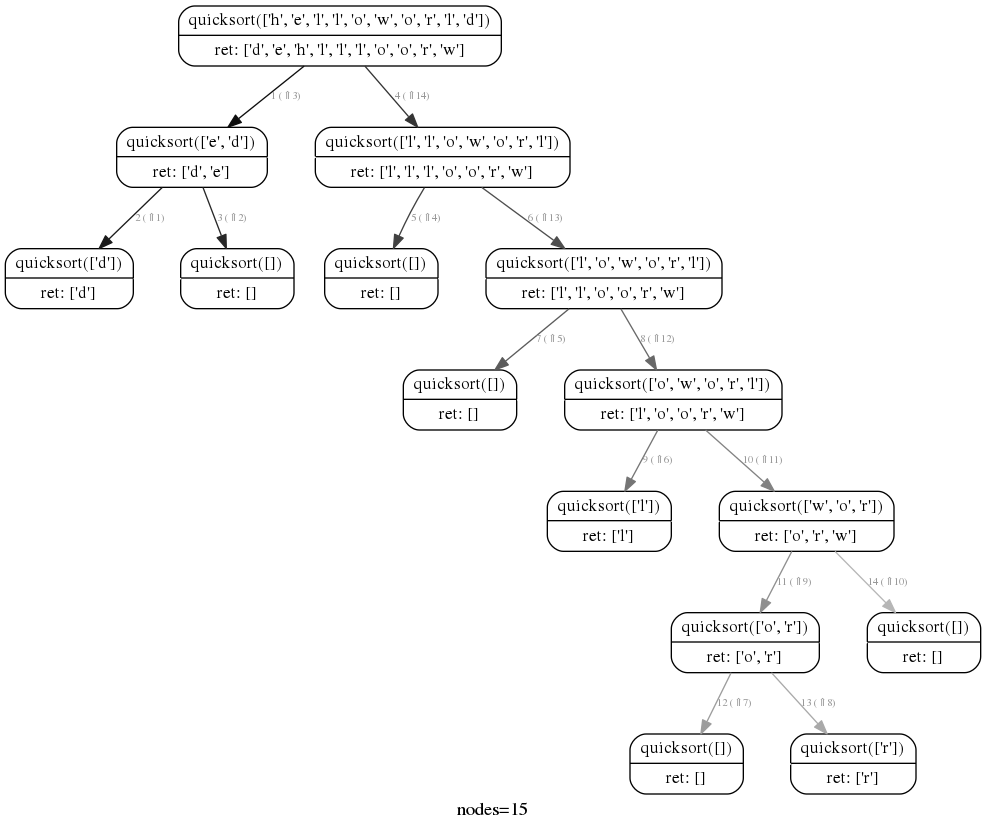# roza / rcviz

Python call graph visualization for recursive functions.

Geek Repo

Github PK Tool

# rcviz

• Python module to visualize a recursion as a tree with arguments and return values at each node.
• Provides a decorator to instrument target functions (as opposed to trace or debugger based approaches)
• Uses pygraphviz to render the graph.

## usage

1. Use the @viz decorator to instrument the recursive function.

@viz
def factorial(n):

1. Render the recursion with

callgraph.render("outfile.png")

The output file type is derived from the file name. Supported types include .dot (graphviz dot file), .png (png image), .svg (vector graphic)

## example

Output for recursive Fibonacci function and for a Recursive Descent parse can be found on this blog post

```from rcviz import callgraph, viz

@viz
def quicksort(items):
if len(items) <= 1:
return items
else:
pivot = items
lesser = quicksort([x for x in items[1:] if x < pivot])
greater = quicksort([x for x in items[1:] if x >= pivot])
return lesser + [pivot] + greater

print quicksort( list("helloworld") )
callgraph.render("sort.png")```

## outputNote:

1. The edges are numbered by the order in which they were traversed by the execution.
2. The edges are colored from black to grey to indicate order of traversal : black edges first, grey edges last.

Experimental

Show intermediate values of local variables in the output render by invoking decoratedfunction.track(param1=val1, param2=val2,...). In the quicksort example above you can track the pivot with:

```	pivot = items
quicksort.track(the_pivot=pivot) # shows a new row labelled the_pivot in each node ```

## dependencies

This requires the native graphviz and libgraphviz-dev packages pre-installed. e.g. On ubuntu do:

\$sudo apt-get install graphviz libgraphviz-dev

then

For python2:

python -m virtualenv .venv source .venv/bin/activate python setup.py install

For python3.x:

python -m venv .venv source .venv/bin/activate python setup.py install

Tested on python 2.7.3 and python 3.6